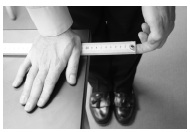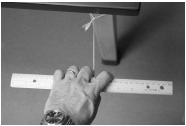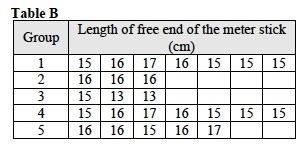# Investigation 1 – Lab### MINDSET

This Investigation is designed to:

• demonstrate to you that the back and forth movement of objects are the vibrations that create sound,
• allow you to create sounds by causing matter to vibrate in standing waves,
• allow you to experience how vibrations and the resulting sounds can be transferred from one object to another,
• demonstrate that vibrations consist of nodes and antinodes,
• demonstrate to you the relationship between wavelength and frequency, and
• allow you to create sounds in a recognizable way.

### BE PREPARED

Student Preparation for the Investigation includes gathering the following materials.

Note: The materials are listed in your SDR. They are also listed below for your reference.

• (1) meter stick
• (1) metric ruler
• (1) length of string one meter long
• (1) lab marker

Direct one student from each lab group to collect the materials listed in their SDRs.

### INVESTIGATE

• All matter is able to vibrate and that these vibrations are what cause sound.
• In this Investigation, you will demonstrate the nature of the vibrations that cause sound.
• Reflect on the PreLab video as you move through the procedural steps.
• During the Experiment, every procedural step is important. If one step is skipped, data can become invalid. To help you keep on track, read each step thoroughly, complete the step, then check it off (Read it – Do it – Check it).
• Complete all of the procedural steps in your SDR.

Note: The procedural steps are listed below for your reference.

Trial 1:

1. Place the meter stick on the lab table with the first 10 cm (from the 0 mark to the 10 cm mark) extending over the edge.
2. One group member should place their hand on the meter stick at the 10 cm mark and press down firmly.
3. Another group member should grasp the free end of the meter stick and bend it up slightly. They should then let go so that the meter stick vibrates.1. Observe the motion of the free end of the meter stick as it vibrates. If necessary, repeat the procedure. Record your observations in Table A.
2. Repeat the same procedure with 20, 30, 40, 50, and 60 cm of the meter stick extending over the edge of the table. If necessary, repeat the procedure for each length. Record your observations in Table A.
3. Answer the following questions:
1. As you increased the length the meter stick extended over the edge of the table from 20 cm to 80 cm, did the meter stick vibrate more slowly or more rapidly?
2. As you increased the length of the meter stick further over the edge of the table did the frequency of the vibrations increase or decrease?
3. When the meter stick was extended only 10 cm over the edge of the table why do you think that it was difficult to see the vibrations?
4. As you increased the length the meter stick extended over the edge of the table, why did it vibrate with lower frequencies?

Trial 2:

1. In this Trial, you will analyze the frequencies and wavelengths of different standing waves in vibrating lengths of string.
2. Tie one end of the string around the top of one of the legs of the lab table.
3. Using the meter stick, measure 10cm from the knot that you used to tie the string. Mark the 10cm length on the string with the lab marker.
4. Measure and mark the string 20, 30, 40, and 50 cm from the knot.
5. Wrap the free end of the string around the center of the metric ruler so that 50cm of the string extends from the knot to the ruler. The 50 cm mark on the string should be close to the edge of the ruler.
6. Grasp the ruler in the center with your fingers on either side of the string but not touching it. Pull on the string and pluck or strum the string with the fingers of your other hand.1. All members of the lab group should observe the motion of the string and listen to the sound.
2. Continue wrapping the free end of the string around the center of the metric ruler so that 40 cm of string extends from the knot to the ruler. The 40 cm mark on the string should be close to the edge of the ruler.
3. Grasp the center of the ruler with your fingers on either side of the string but not touching it. Pull on the string with the same amount of force that you used before. Pluck the string with the fingers of your other hand.
4. All members of the lab group should observe the motion of the string and listen to the sound.
5. Repeat the same procedure for the 30, 20, and 10 cm lengths of string. Remember to pull on the string with the same amount of force.
6. Repeat the procedure for each length so every group member takes a turn.
7. Calculate the wavelength of each wave by multiplying the length of each length of string by 2. Record the wavelengths in Table B.
8. Answer the following questions.
1. As you decreased the wavelength of the standing wave did the string vibrate more slowly or more rapidly?
2. As you decreased the wavelength of the standing wave did the frequency of the vibrations increase or decrease?
3. As you decreased the wavelength of the standing wave, why did it vibrate with higher frequencies?
4. In Trial 1 you used different lengths of a vibrating meter stick to generate sounds of different frequencies. In Trial 2, you used different lengths of string to generate sounds of different frequencies. What is the relationship between the wavelength of the standing wave in the meter stick and the length it extended over the edge of the table?

Trial 3:

1. In this Trial, you will analyze a sequence of sounds generated by a vibrating meter stick.
2. Place the meter stick on the lab table, with the first 15 cm (from the 0 mark to the 15 cm mark) extending over the edge. Place one hand on the meter stick at the 15 cm mark and press down.
3. Move the meter stick so that different lengths of it extend over the edge of the lab table. You will use the sequence of lengths shown in Table B.1. There are five groups of lengths. There are three, five, or seven lengths in each group. Read the sequence of lengths from left to right starting with Group 1 and then go to Group 2, Group 3, etc., until you have finished with Group 5. Pause after you have finished each Group.
2. For each length in Table B, pluck the free end of the meter stick once.
3. As you pluck the meter stick, listen to the sequence of frequencies and try to determine what song you are playing.
4. What song did you just play?

### CLEAN UP

Be sure to clean up your bench after you have finished your experiments.